International Tables for Crystallography (2006). Vol. B. ch. 1.2, pp. 10-24   | 1 | 2 | https://doi.org/10.1107/97809553602060000550

## Contents

• 1.2. The structure factor  (pp. 10-24)
• 1.2.1. Introduction  (p. 10) | html | pdf |
• 1.2.2. General scattering expression for X-rays  (p. 10) | html | pdf |
• 1.2.3. Scattering by a crystal: definition of a structure factor  (p. 10) | html | pdf |
• 1.2.4. The isolated-atom approximation in X-ray diffraction  (pp. 10-11) | html | pdf |
• 1.2.5. Scattering of thermal neutrons  (p. 11) | html | pdf |
• 1.2.5.1. Nuclear scattering  (p. 11) | html | pdf |
• 1.2.5.2. Magnetic scattering  (p. 11) | html | pdf |
• 1.2.6. Effect of bonding on the atomic electron density within the spherical-atom approximation: the kappa formalism  (pp. 11-13) | html | pdf |
• 1.2.7. Beyond the spherical-atom description: the atom-centred spherical harmonic expansion  (pp. 14-15) | html | pdf |
• 1.2.7.1. Direct-space description of aspherical atoms  (pp. 14-15) | html | pdf |
• 1.2.7.2. Reciprocal-space description of aspherical atoms  (p. 15) | html | pdf |
• 1.2.8. Fourier transform of orbital products  (pp. 17-18) | html | pdf |
• 1.2.8.1. One-centre orbital products  (p. 18) | html | pdf |
• 1.2.8.2. Two-centre orbital products  (p. 18) | html | pdf |
• 1.2.9. The atomic temperature factor  (p. 18) | html | pdf |
• 1.2.10. The vibrational probability distribution and its Fourier transform in the harmonic approximation  (pp. 18-19) | html | pdf |
• 1.2.11. Rigid-body analysis  (pp. 19-22) | html | pdf |
• 1.2.12. Treatment of anharmonicity  (pp. 22-23) | html | pdf |
• 1.2.12.1. The Gram–Charlier expansion  (p. 22) | html | pdf |
• 1.2.12.2. The cumulant expansion  (pp. 22-23) | html | pdf |
• 1.2.12.3. The one-particle potential (OPP) model  (p. 23) | html | pdf |
• 1.2.12.4. Relative merits of the three expansions  (p. 23) | html | pdf |
• 1.2.13. The generalized structure factor  (pp. 23-24) | html | pdf |
• 1.2.14. Conclusion  (p. 24) | html | pdf |
• References | html | pdf |
• Tables
• Table 1.2.7.1. Real spherical harmonic functions ( x , y , z are direction cosines)  (pp. 12-13) | html | pdf |
• Table 1.2.7.2. Index-picking rules of site-symmetric spherical harmonics (Kara & Kurki-Suonio, 1981)  (p. 15) | html | pdf |
• Table 1.2.7.3. `Kubic Harmonic' functions  (pp. 16-17) | html | pdf |
• Table 1.2.7.4. Closed-form expressions for Fourier transform of Slater-type functions (Avery & Watson, 1977; Su & Coppens, 1990)  (p. 19) | html | pdf |
• Table 1.2.8.1. Products of complex spherical harmonics as defined by equation (1.2.7.2 a )  (p. 20) | html | pdf |
• Table 1.2.8.2. Products of real spherical harmonics as defined by equations (1.2.7.2 b ) and (1.2.7.2 c )  (p. 20) | html | pdf |
• Table 1.2.8.3. Products of two real spherical harmonic functions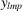in terms of the density functions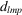defined by equation (1.2.7.3 b )  (p. 21) | html | pdf |
• Table 1.2.11.1. The arrays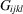and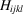to be used in the observational equations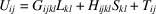[equation (1.2.11.9)]  (p. 21) | html | pdf |
• Table 1.2.12.1. Some Hermite polynomials (Johnson & Levy, 1974; Zucker & Schulz, 1982)  (p. 22) | html | pdf |